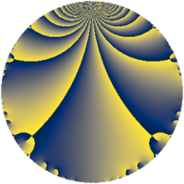# Properties

 Label 4830.2.a.bvLevel $4830$ Weight $2$ Character orbit 4830.a Self dual yes Analytic conductor $38.568$ Analytic rank $0$ Dimension $2$ CM no Inner twists $1$

# Related objects

## Newspace parameters

 Level: $$N$$ $$=$$ $$4830 = 2 \cdot 3 \cdot 5 \cdot 7 \cdot 23$$ Weight: $$k$$ $$=$$ $$2$$ Character orbit: $$[\chi]$$ $$=$$ 4830.a (trivial)

## Newform invariants

 Self dual: yes Analytic conductor: $$38.5677441763$$ Analytic rank: $$0$$ Dimension: $$2$$ Coefficient field: $$\Q(\sqrt{33})$$ Defining polynomial: $$x^{2} - x - 8$$ Coefficient ring: $$\Z[a_1, \ldots, a_{29}]$$ Coefficient ring index: $$2$$ Twist minimal: yes Fricke sign: $$-1$$ Sato-Tate group: $\mathrm{SU}(2)$

## $q$-expansion

Coefficients of the $$q$$-expansion are expressed in terms of $$\beta = \sqrt{33}$$. We also show the integral $$q$$-expansion of the trace form.

 $$f(q)$$ $$=$$ $$q + q^{2} + q^{3} + q^{4} - q^{5} + q^{6} - q^{7} + q^{8} + q^{9} +O(q^{10})$$ $$q + q^{2} + q^{3} + q^{4} - q^{5} + q^{6} - q^{7} + q^{8} + q^{9} - q^{10} -2 q^{11} + q^{12} - q^{14} - q^{15} + q^{16} + 2 q^{17} + q^{18} - q^{20} - q^{21} -2 q^{22} + q^{23} + q^{24} + q^{25} + q^{27} - q^{28} + ( 3 + \beta ) q^{29} - q^{30} + ( 3 + \beta ) q^{31} + q^{32} -2 q^{33} + 2 q^{34} + q^{35} + q^{36} + ( 3 - \beta ) q^{37} - q^{40} + 6 q^{41} - q^{42} + ( 3 - \beta ) q^{43} -2 q^{44} - q^{45} + q^{46} + ( 2 - 2 \beta ) q^{47} + q^{48} + q^{49} + q^{50} + 2 q^{51} + ( -1 - \beta ) q^{53} + q^{54} + 2 q^{55} - q^{56} + ( 3 + \beta ) q^{58} + ( 9 - \beta ) q^{59} - q^{60} -2 q^{61} + ( 3 + \beta ) q^{62} - q^{63} + q^{64} -2 q^{66} + ( 1 + \beta ) q^{67} + 2 q^{68} + q^{69} + q^{70} + ( 9 + \beta ) q^{71} + q^{72} + ( 7 - \beta ) q^{73} + ( 3 - \beta ) q^{74} + q^{75} + 2 q^{77} + ( -1 + \beta ) q^{79} - q^{80} + q^{81} + 6 q^{82} + ( -2 + 2 \beta ) q^{83} - q^{84} -2 q^{85} + ( 3 - \beta ) q^{86} + ( 3 + \beta ) q^{87} -2 q^{88} + ( -5 + \beta ) q^{89} - q^{90} + q^{92} + ( 3 + \beta ) q^{93} + ( 2 - 2 \beta ) q^{94} + q^{96} + ( -1 + \beta ) q^{97} + q^{98} -2 q^{99} +O(q^{100})$$ $$\operatorname{Tr}(f)(q)$$ $$=$$ $$2q + 2q^{2} + 2q^{3} + 2q^{4} - 2q^{5} + 2q^{6} - 2q^{7} + 2q^{8} + 2q^{9} + O(q^{10})$$ $$2q + 2q^{2} + 2q^{3} + 2q^{4} - 2q^{5} + 2q^{6} - 2q^{7} + 2q^{8} + 2q^{9} - 2q^{10} - 4q^{11} + 2q^{12} - 2q^{14} - 2q^{15} + 2q^{16} + 4q^{17} + 2q^{18} - 2q^{20} - 2q^{21} - 4q^{22} + 2q^{23} + 2q^{24} + 2q^{25} + 2q^{27} - 2q^{28} + 6q^{29} - 2q^{30} + 6q^{31} + 2q^{32} - 4q^{33} + 4q^{34} + 2q^{35} + 2q^{36} + 6q^{37} - 2q^{40} + 12q^{41} - 2q^{42} + 6q^{43} - 4q^{44} - 2q^{45} + 2q^{46} + 4q^{47} + 2q^{48} + 2q^{49} + 2q^{50} + 4q^{51} - 2q^{53} + 2q^{54} + 4q^{55} - 2q^{56} + 6q^{58} + 18q^{59} - 2q^{60} - 4q^{61} + 6q^{62} - 2q^{63} + 2q^{64} - 4q^{66} + 2q^{67} + 4q^{68} + 2q^{69} + 2q^{70} + 18q^{71} + 2q^{72} + 14q^{73} + 6q^{74} + 2q^{75} + 4q^{77} - 2q^{79} - 2q^{80} + 2q^{81} + 12q^{82} - 4q^{83} - 2q^{84} - 4q^{85} + 6q^{86} + 6q^{87} - 4q^{88} - 10q^{89} - 2q^{90} + 2q^{92} + 6q^{93} + 4q^{94} + 2q^{96} - 2q^{97} + 2q^{98} - 4q^{99} + O(q^{100})$$

## Embeddings

For each embedding $$\iota_m$$ of the coefficient field, the values $$\iota_m(a_n)$$ are shown below.

For more information on an embedded modular form you can click on its label.

Label $$\iota_m(\nu)$$ $$a_{2}$$ $$a_{3}$$ $$a_{4}$$ $$a_{5}$$ $$a_{6}$$ $$a_{7}$$ $$a_{8}$$ $$a_{9}$$ $$a_{10}$$
1.1
 −2.37228 3.37228
1.00000 1.00000 1.00000 −1.00000 1.00000 −1.00000 1.00000 1.00000 −1.00000
1.2 1.00000 1.00000 1.00000 −1.00000 1.00000 −1.00000 1.00000 1.00000 −1.00000
 $$n$$: e.g. 2-40 or 990-1000 Significant digits: Format: Complex embeddings Normalized embeddings Satake parameters Satake angles

## Atkin-Lehner signs

$$p$$ Sign
$$2$$ $$-1$$
$$3$$ $$-1$$
$$5$$ $$1$$
$$7$$ $$1$$
$$23$$ $$-1$$

## Inner twists

This newform does not admit any (nontrivial) inner twists.

## Twists

By twisting character orbit
Char Parity Ord Mult Type Twist Min Dim
1.a even 1 1 trivial 4830.2.a.bv 2

By twisted newform orbit
Twist Min Dim Char Parity Ord Mult Type
4830.2.a.bv 2 1.a even 1 1 trivial

## Hecke kernels

This newform subspace can be constructed as the intersection of the kernels of the following linear operators acting on $$S_{2}^{\mathrm{new}}(\Gamma_0(4830))$$:

 $$T_{11} + 2$$ $$T_{13}$$ $$T_{17} - 2$$ $$T_{19}$$ $$T_{29}^{2} - 6 T_{29} - 24$$

## Hecke characteristic polynomials

$p$ $F_p(T)$
$2$ $$( -1 + T )^{2}$$
$3$ $$( -1 + T )^{2}$$
$5$ $$( 1 + T )^{2}$$
$7$ $$( 1 + T )^{2}$$
$11$ $$( 2 + T )^{2}$$
$13$ $$T^{2}$$
$17$ $$( -2 + T )^{2}$$
$19$ $$T^{2}$$
$23$ $$( -1 + T )^{2}$$
$29$ $$-24 - 6 T + T^{2}$$
$31$ $$-24 - 6 T + T^{2}$$
$37$ $$-24 - 6 T + T^{2}$$
$41$ $$( -6 + T )^{2}$$
$43$ $$-24 - 6 T + T^{2}$$
$47$ $$-128 - 4 T + T^{2}$$
$53$ $$-32 + 2 T + T^{2}$$
$59$ $$48 - 18 T + T^{2}$$
$61$ $$( 2 + T )^{2}$$
$67$ $$-32 - 2 T + T^{2}$$
$71$ $$48 - 18 T + T^{2}$$
$73$ $$16 - 14 T + T^{2}$$
$79$ $$-32 + 2 T + T^{2}$$
$83$ $$-128 + 4 T + T^{2}$$
$89$ $$-8 + 10 T + T^{2}$$
$97$ $$-32 + 2 T + T^{2}$$